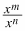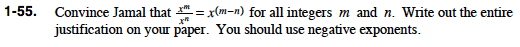### Home > PC > Chapter 1 > Lesson 1.1.4 > Problem1-55

1-55.

Convince Jamal that= x (mn) for all integers m and n. Write out the entire justification on your paper. You should use negative exponents. Homework Help ✎Here is the first step. Continue one step at a time until the right side of the equation matches the right side in the problem.

$\frac{x^m}{x^n} = x^m \cdot x^{-n}$